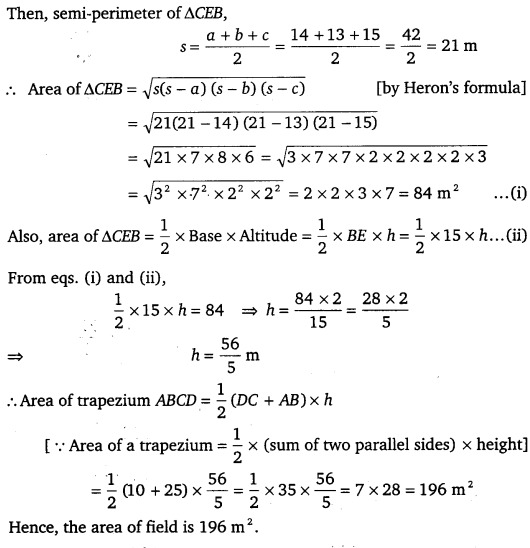# CLASS 9 MATH NCERT SOLUTION FOR CHAPTER – 12 HERON’S FORMULA EX – 12.2

## Heron’s Formula

Question 1.
A park in the shape of a quadrilateral ABCD has ∠C = 90°, AB = 9 m, BC = 12 m, CD = 5 m and AD = 8 m. How much area does it occupy?

Solution:
Given, quadrilateral ABCD in which AB = 9 m, BC = 12 m, CD = 5 m, AD = 8 m and ∠C = 90°. Now, join the diagonal BD which divides quadrilateral ABCD into two triangles.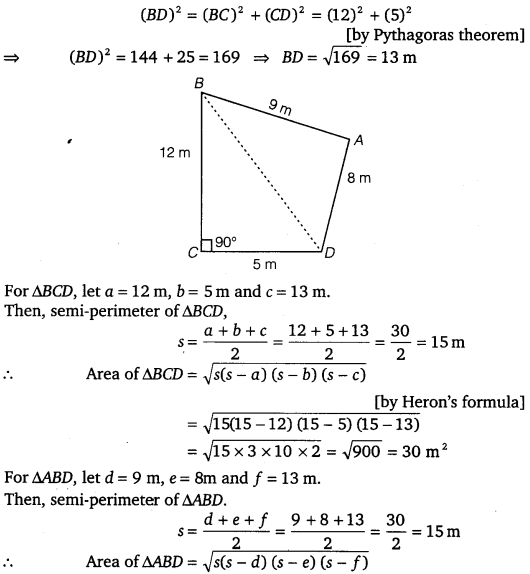Question 2.
Find the area of a quadrilateral ABCD in which AB = 3 cm, BC = 4 cm, CD 4 cm, DA = 5 cm and
AC = 5 cm.

Solution:
Area of quadrilateral ABCD = Area of Δ ABC + Area of Δ ACD
In Δ ABC,
We have, AB = 3 cm, BC = 4 cm, CA = 5 cm
Therefore, AB2 + BC2 = 32 + 42 = 9 +16 = 25 = (5)2 = CA2

Question 3.
Radha made a picture of an aeroplane with coloured paper as shown in figure. Find the total area of the paper used.

Solution:
For region I (triangle),
Let a = 5 cm, b = 5 cm and c = 1 cm
Then, semi-perimeter of I triangle$s=\cfrac { a+b+c }{ 2 } =\cfrac { 5+5+1 }{ 2 } =\cfrac { 11 }{ 2 } cm$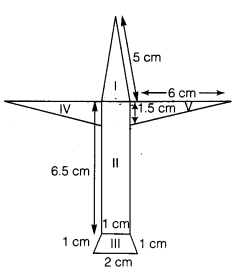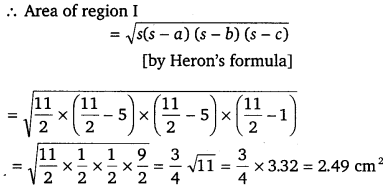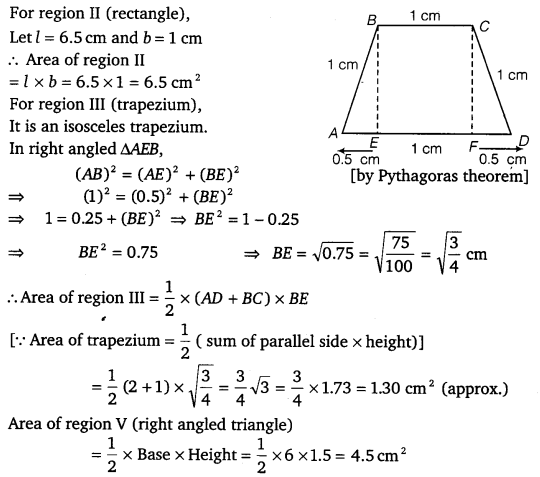Similarly area of region IV (right angled triangle )
= 4.5 cm2 [Since, it has same dimensions as region V]
∴ Total area of paper used = Area of region I + Area of region II + Area of region III +Area of region IV + Area of region V
= 2.49 + 6.5 +1. 30 + 4.5 + 4.5= 19.29 = 19.3 cm2 (approx.)

Question 4.
A triangle and a parallelogram have the same base and the same area. If the sides of the triangle are 26 cm, 28 cm and 30 cm and the parallelogram stands on the base 28 cm, find the height of the parallelogram.

Solution:
Let ABC be a triangle with sides
AB = 26 cm, BC = 28 cm, CA = 30 cm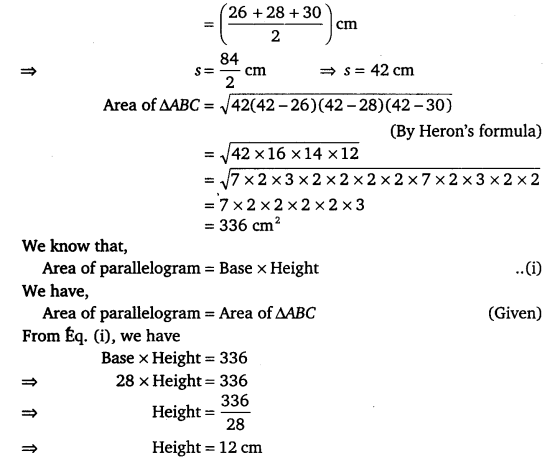Question 5.
A rhombus shaped field has green grass for 18 cows to graze. If each side of the rhombus is 30 m and its longer diagonal is 48 m, how much area of grass field will each cow be getting?

Solution:
Given, ABCD is a rhombus shaped field whose each side is 30 m and longer diagonal AC is 48 m.Clearly, the diagonal AC divides the rhombus into two triangles, Δ ABC and Δ ADC which are congruent.
Area of Δ ABC = Area of Δ ADC
Also, Δ ABC and Δ ADC have equal perimeters.
Now, semi-perimeter of Δ ABC,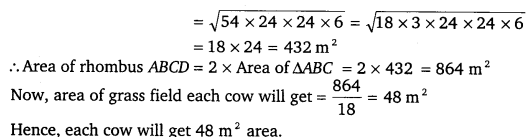Question 6.
An umbrella is made by stitching 10 triangular pieces of cloth of two different colors, each piece measuring 20 cm, 50 cm, and 50 cm. How much cloth of each color is required for the umbrella?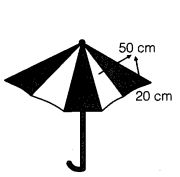Solution:
Let given sides of one triangular piece of cloth be a = 20 cm, b = 50 cm and c = 50 cm.
Then, semi-perimeter of one triangular piece.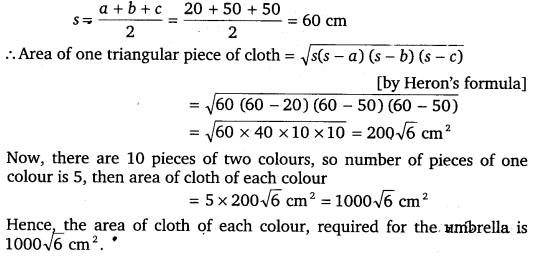Question 7.
A kite in the shape of a square with a diagonal 32 cm and an isosceles triangle of base 8 cm and sides 6 cm, each is to be made of three different shades as shown in the figure. How much paper of each shade has been used in it?

Solution:Given, sides of ADEF are DE = DF = 6 cm and EF = 8 cm.
Also, diagonal of square ABCD = 32 cm
We know that diagonals of a square bisect each other at right angle.
OA = OB = OC = OD =$\cfrac { 32 }{ 2 }$ = 16 cm
Now, area of region I = 2 x Area of AAOB = 2 x$\cfrac { 1 }{ 2 }$ x OA x OB
[∵ area of right angled triangle =$\cfrac { 1 }{ 2 }$ x base x height]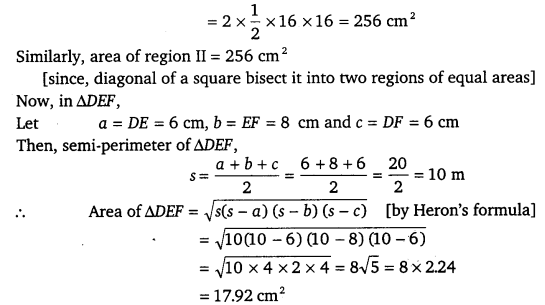Hence, the area of paper of I color used in making kite = 256 cmArea of paper of II color used in making kite = 256 cmand area of paper of III color used in making kite = 17.92 cm2

Question 8.
A floral design on a floor is made up of 16 tiles, which are triangular, the sides of the triangle being 28 cm, 9 cm and 35 cm, Find the cost of polishing the tiles at the rate of 50 paise per cm2.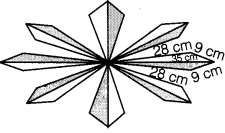Solution:
Given, length of the side of the triangular tile are 28 cm, 9 cm, and 35 cm.
Let a = 28 cm, b = 9 cm and c = 35 cm
Then, semi-perimeter of one triangular tile,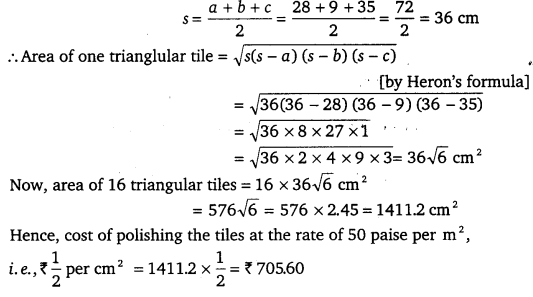Question 9.
A field is in the shape of a trapezium, whose parallel sides are 25 m and 10 m and the non-parallel sides are 14 m and 13 m. Find the area of the field.

Solution:Let ABCD be the given field in the form of a trapezium in which AB = 25 m and CD = 10 m, BC = 13 m, AD = 14 m and DC || AB.
Through C, draw CE||DA and let it meets AB at E.
Let CP h m be the height of the trapezium.
Now, DC || AE     [∵ DC 11 AB given]
and  CE||DA       [by construction]
Thus, AECD is a parallelogram.
⇒ AE = DC = 10 m and CE = DA = 14 m
In A CEB, we have, CB = 13m, CE = 14 m
and BE = AB – AE = 25 -10 = 15 m
Let a = 14 m, b = 13 m and c = 15 m